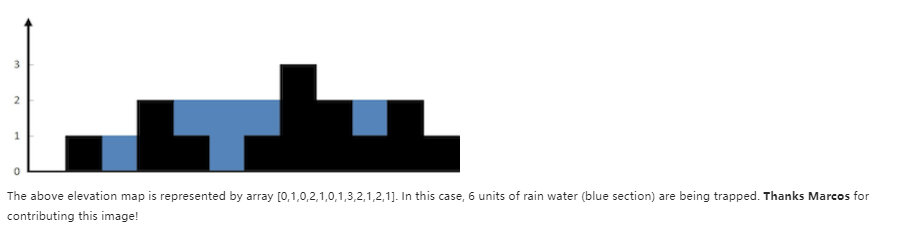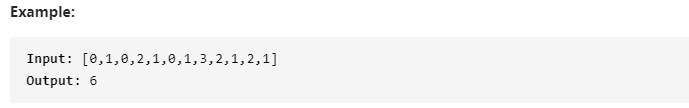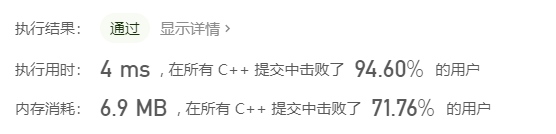# 【小白爬Leetcode42】8.5 接雨水 Trapping Rain Water

### 【小白爬Leetcode42】8.5 接雨水 Trapping Rain Water

Leetcode 42 h a r d \color{#FF0000}{hard}

# 题目

## Discription

Given n non-negative integers representing an elevation map where the width of each bar is 1, compute how much water it is able to trap after raining.# 思路一 栈实现

class Solution {
public:
int trap(vector<int>& height) {
int len = height.size();
if(len<3) return 0;
stack<int> s;
int ans = 0, index = 0;
while(index<len){
while(!s.empty()&&height[index]>height[s.top()]){
int smallest_height = height[s.top()];
s.pop();
if(s.empty()) break; //如果此时栈空，则退出while循环
int depth = min(height[index],height[s.top()]) -smallest_height;
int width = index-s.top()-1;
ans += depth*width;
}
s.push(index++);
}
return ans;
}
};


# 思路二 动态规划（很好理解）

class Solution {
public:
int trap(vector<int>& height) {
int len = height.size();
if(len<3) return 0;
vector<int> left_max(len,0);
left_max = height;
vector<int> right_max(len,0);
right_max[len-1] = height[len-1];
for(int i=1;i<len;i++){
left_max[i] = max(left_max[i-1],height[i]);
}
for(int i=len-2;i>=0;i--){
right_max[i] = max(right_max[i+1],height[i]);
}
int ans = 0;
for(int i=1;i<len-1;i++){
ans += min(left_max[i],right_max[i])-height[i];
}
return ans;
}
};


# 思路三 双指针法（只遍历一次，非常巧妙）

• 在计算积水深度的时候，积水深度取决于当前积水深度和min(左/右边的最大深度)，也就是说，只取决于较小的那边，而与较大的那边无关（短板）。
• left_max数组从左向右是一个递增数组，而right_max从右向左是一个递增数组。

1. 设置一个int left=0指针和一个int right = height.size()-1指针。

2. 记录目前左边的最高的位置int left_max = 0和右边最高的位置int right_max = 0

3. height[left]<height[right]的时候，分两种情况：
【a】 如果height[left] >= left_max，说明此格不会产生积水，否则会从左边漏掉。那么只要更新left_max即可。
【b】 如果height[left] < left_max，说明当前元素height[left]是低洼处，且积水深度取决于left_max ，为什么？因为这里有一个隐含信息left_max恒小于height[right]。试思考，left_max的值只可能是从更左边的位置得来的，且每次更新left_max是在height[left]<height[right]的大前提下，因此 left_max恒小于height[right]

那么判断是否能积水，不是要根据min(left_max[i],right_max[i])来判断吗？是的，这里还有一个隐含信息就是：left_max不仅恒小于height[right]（小王），更会小于右边的最高点（大王）。 因为right_max是从右向左递增的。

综上 积水深度取决于left_max

4. 右边的情况和左边是对称的，因此代码如下：

class Solution {
public:
int trap(vector<int>& height){
int len = height.size();
if(len<3) return 0;
int left = 0;
int right = len-1;
int left_max = 0;
int right_max = 0;
int ans = 0;
while(left<right){
if(height[left]<height[right]){
height[left] >= left_max? left_max=height[left] : ans+=(left_max-height[left]);
left++;
}
else{
height[right] >= right_max? right_max = height[right]: ans+=(right_max-height[right]);
right--;
}
}
return ans;
}
};09-021万+
02-0814112-03732
01-2064
02-2928
05-20536
06-21260
11-12186
03-11205
10-2172